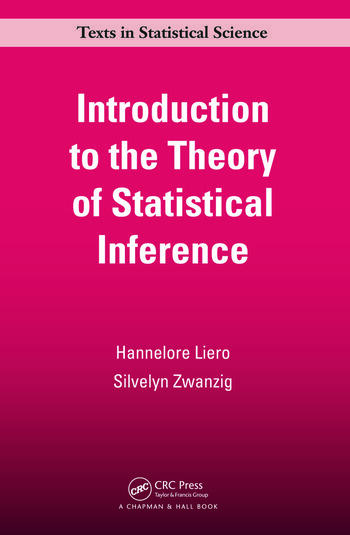# Introduction to the Theory of Statistical Inference

## 1st Edition

Hannelore Liero, Silvelyn Zwanzig

Chapman and Hall/CRC
Published July 20, 2011
Textbook - 284 Pages - 40 B/W Illustrations
ISBN 9781439852927 - CAT# K12437
Series: Chapman & Hall/CRC Texts in Statistical Science

For Instructors Request Inspection Copy

USD\$92.95

FREE Standard Shipping!

## Preview

### Summary

Based on the authors’ lecture notes, Introduction to the Theory of Statistical Inference presents concise yet complete coverage of statistical inference theory, focusing on the fundamental classical principles. Suitable for a second-semester undergraduate course on statistical inference, the book offers proofs to support the mathematics. It illustrates core concepts using cartoons and provides solutions to all examples and problems.

Highlights

• Basic notations and ideas of statistical inference are explained in a mathematically rigorous, but understandable, form
• Classroom-tested and designed for students of mathematical statistics
• Examples, applications of the general theory to special cases, exercises, and figures provide a deeper insight into the material
• Solutions provided for problems formulated at the end of each chapter
• Combines the theoretical basis of statistical inference with a useful applied toolbox that includes linear models
• Theoretical, difficult, or frequently misunderstood problems are marked

The book is aimed at advanced undergraduate students, graduate students in mathematics and statistics, and theoretically-interested students from other disciplines. Results are presented as theorems and corollaries. All theorems are proven and important statements are formulated as guidelines in prose. With its multipronged and student-tested approach, this book is an excellent introduction to the theory of statistical inference.

#### Instructors

We provide complimentary e-inspection copies of primary textbooks to instructors considering our books for course adoption.